# LONCAPA Mathematical Functionality Gerd Kortemeyer Lyman Briggs College

• Slides: 40LON-CAPA Mathematical Functionality Gerd Kortemeyer Lyman Briggs College February 2011LON-CAPA Math Functionality �Today’s Session: somewhat specialized topic �Goal: demonstrate how mathematical assessment is integrated into the LON-CAPA course management functionality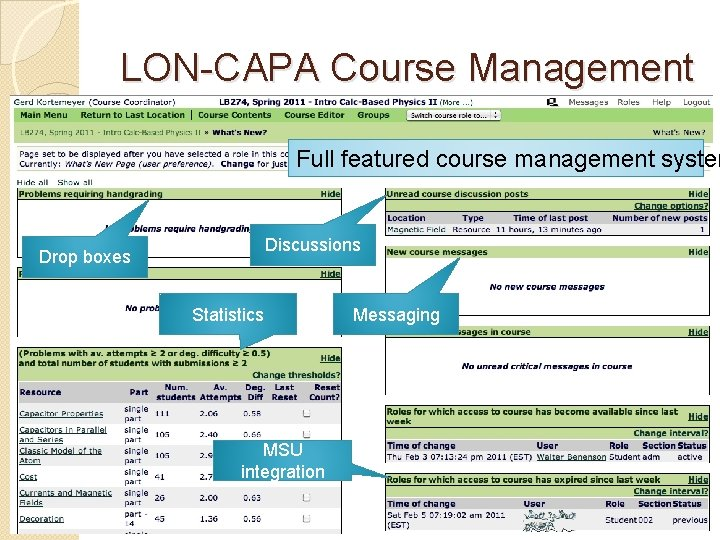LON-CAPA Course Management Full featured course management system Discussions Drop boxes Statistics MSU integration Messaging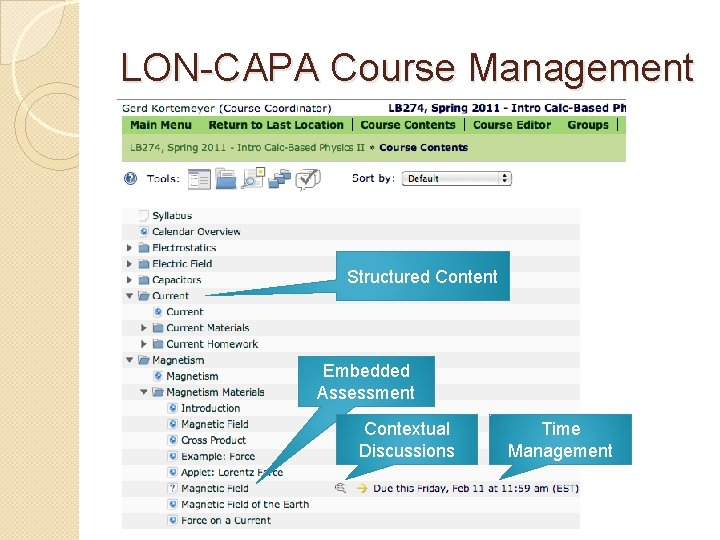LON-CAPA Course Management Structured Content Embedded Assessment Contextual Discussions Time Management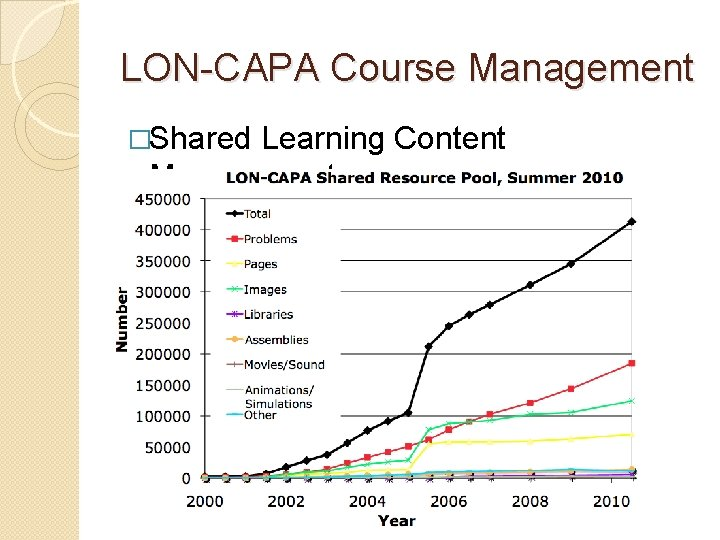LON-CAPA Course Management �Shared Learning Content ManagementParticular Strength: Assessment �Randomized ◦ ◦ ◦ problems: different numbers formulas graphs images options … for each student. �Student can collaborate without “cheating” �Randomized exams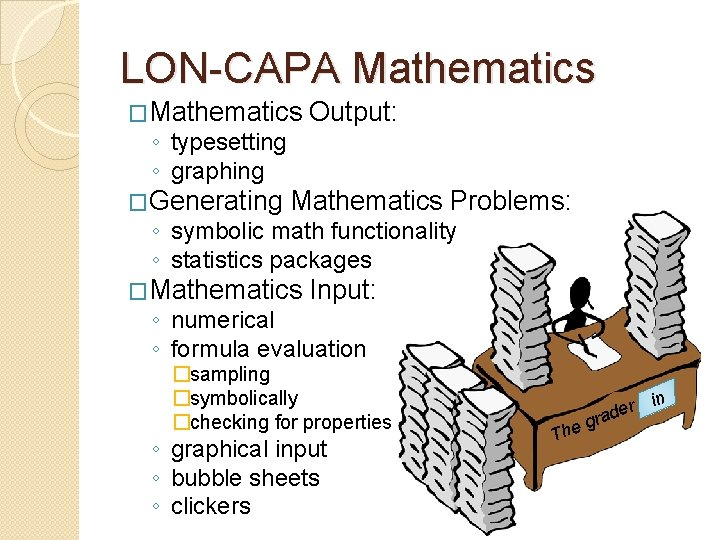LON-CAPA Mathematics �Mathematics ◦ typesetting ◦ graphing �Generating Output: Mathematics Problems: ◦ symbolic math functionality ◦ statistics packages �Mathematics Input: ◦ numerical ◦ formula evaluation �sampling �symbolically �checking for properties ◦ graphical input ◦ bubble sheets ◦ clickers The g er rad is inWARNING Today’s presentation is going to show some very specialized functionality. Because you can does not mean you have to.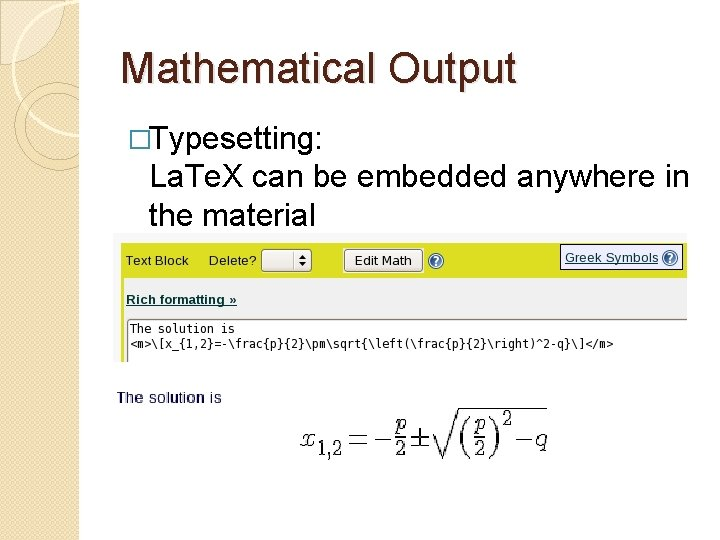Mathematical Output �Typesetting: La. Te. X can be embedded anywhere in the materialMathematical Output �Editor for non-native La. Te. X speakers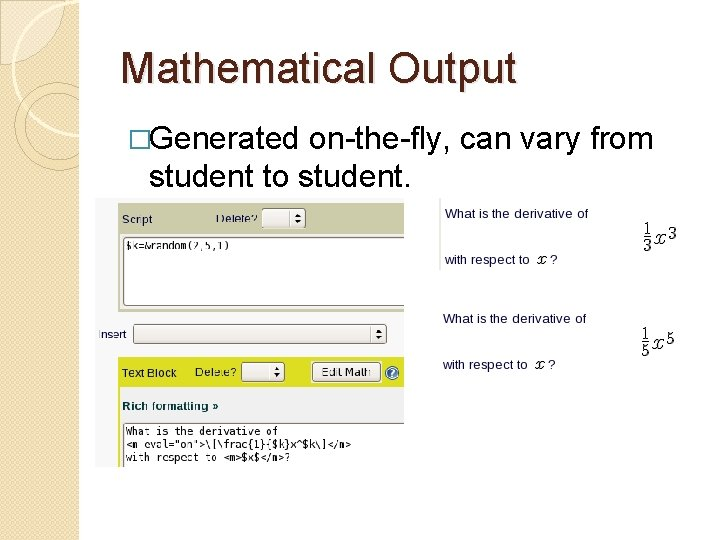Mathematical Output �Generated on-the-fly, can vary from student to student.Mathematical Output �<algebra>-tag to pretty-print the output from computer algebra systems �Example: \$formula=“a*x^5”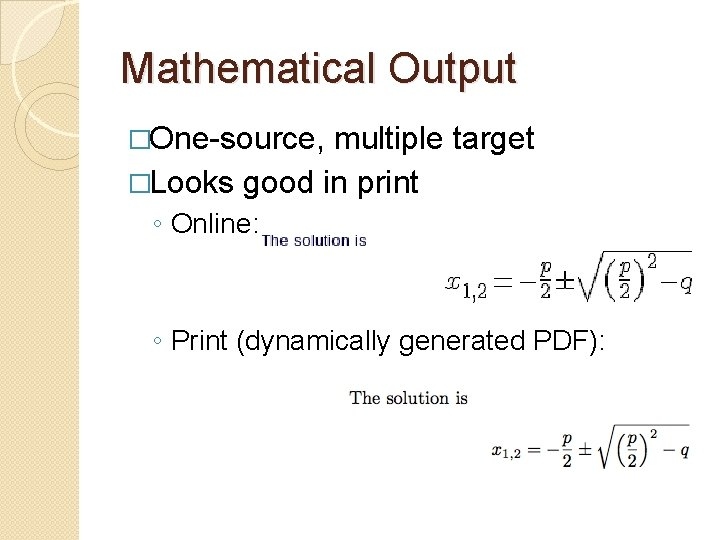Mathematical Output �One-source, multiple target �Looks good in print ◦ Online: ◦ Print (dynamically generated PDF):Mathematical Output �Dynamic Graphing ◦ Data-Points ◦ Functions ◦ Line-Graphics �Internally GNUplot usesMathematical Output �Data points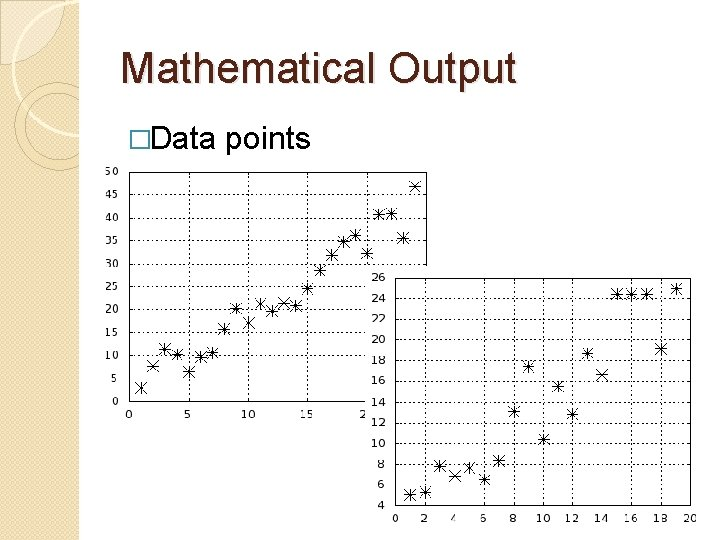Mathematical Output �Data points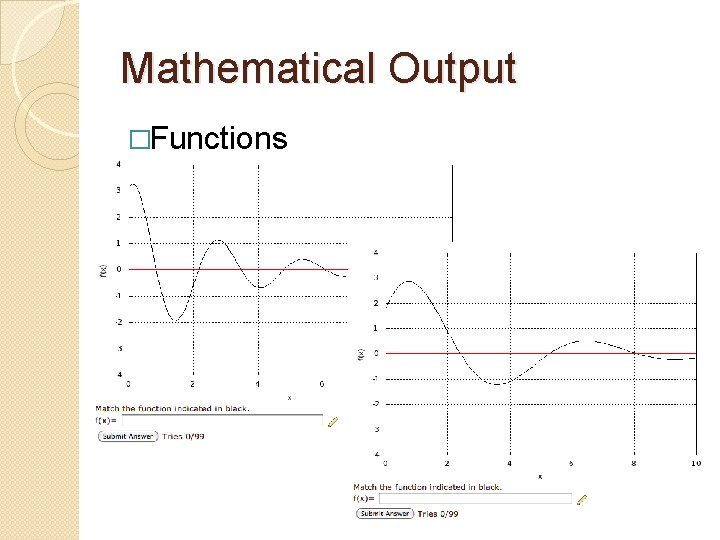Mathematical Output �FunctionsMathematical Output �Line graphics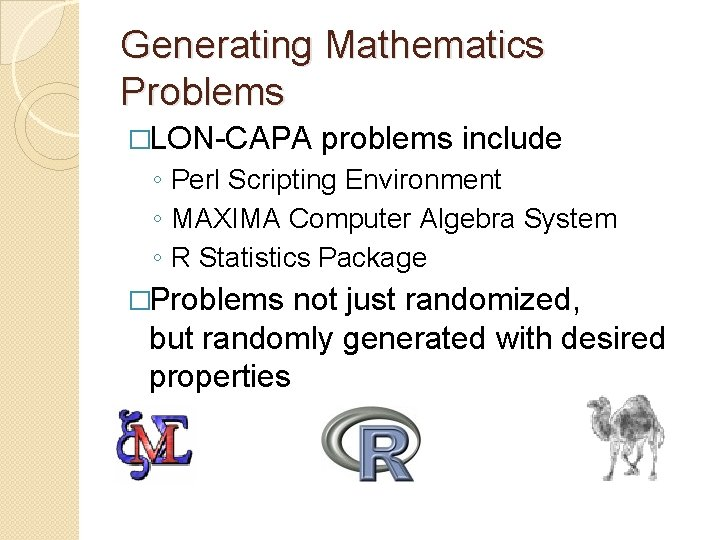Generating Mathematics Problems �LON-CAPA problems include ◦ Perl Scripting Environment ◦ MAXIMA Computer Algebra System ◦ R Statistics Package �Problems not just randomized, but randomly generated with desired propertiesGenerating Mathematics Problems �Direct calls to MAXIMA: \$result=&cas(‘maxima’, \$expression); �Simple example: use computer algebra system to calculate a reduced fraction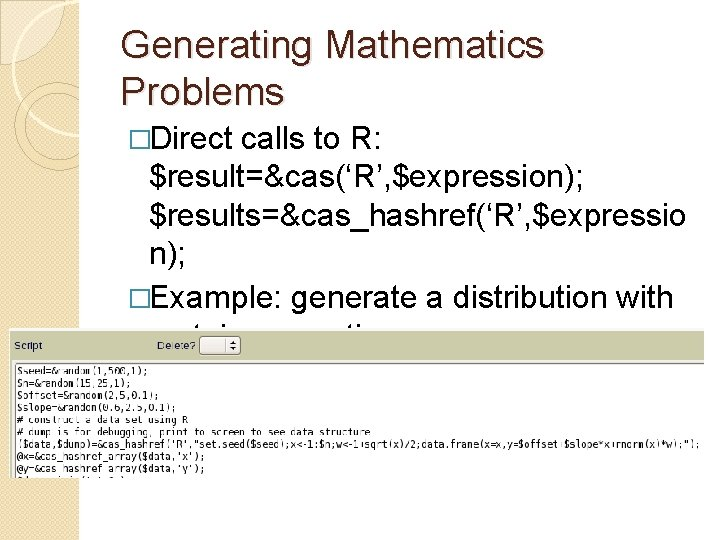Generating Mathematics Problems �Direct calls to R: \$result=&cas(‘R’, \$expression); \$results=&cas_hashref(‘R’, \$expressio n); �Example: generate a distribution with certain properties: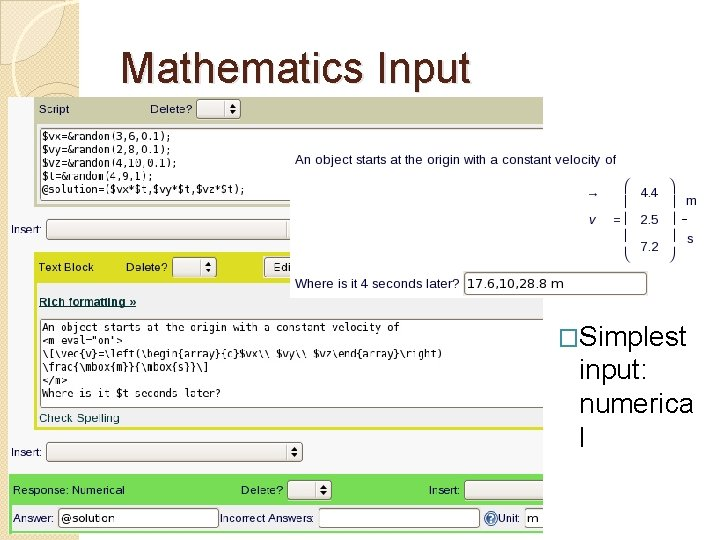Mathematics Input �Simplest input: numerica lMathematics Input � Sampling – approximate function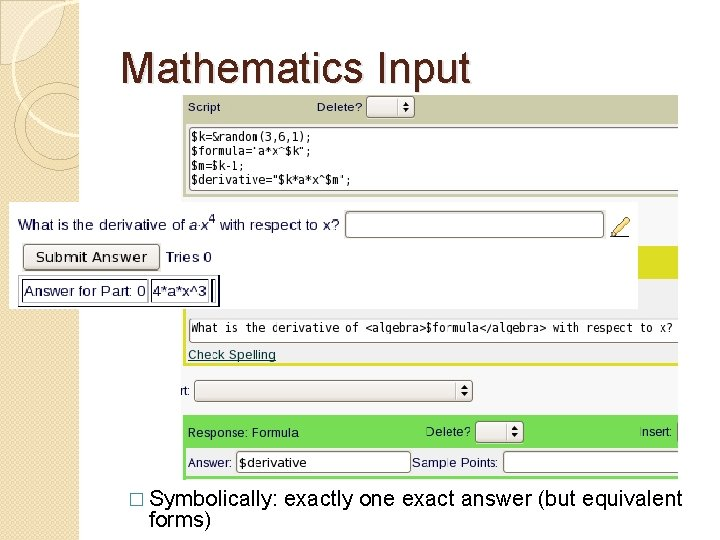Mathematics Input � Symbolically: forms) exactly one exact answer (but equivalent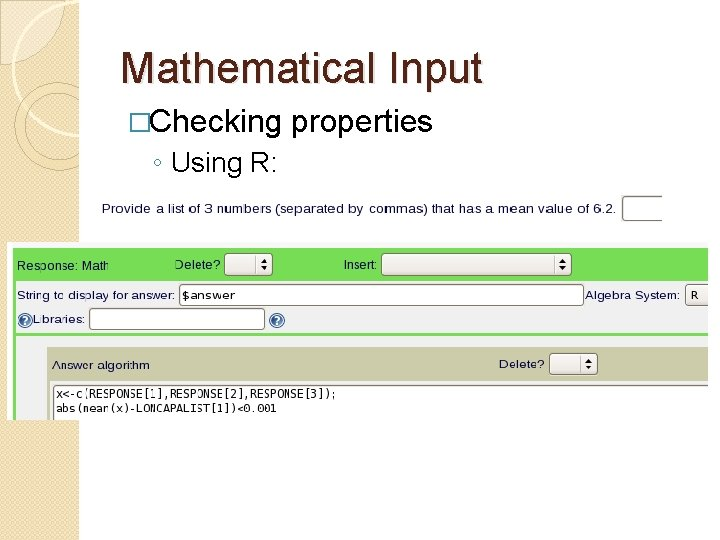Mathematical Input �Checking ◦ Using R: propertiesMathematical Input �Checking properties ◦ Using MAXIMA: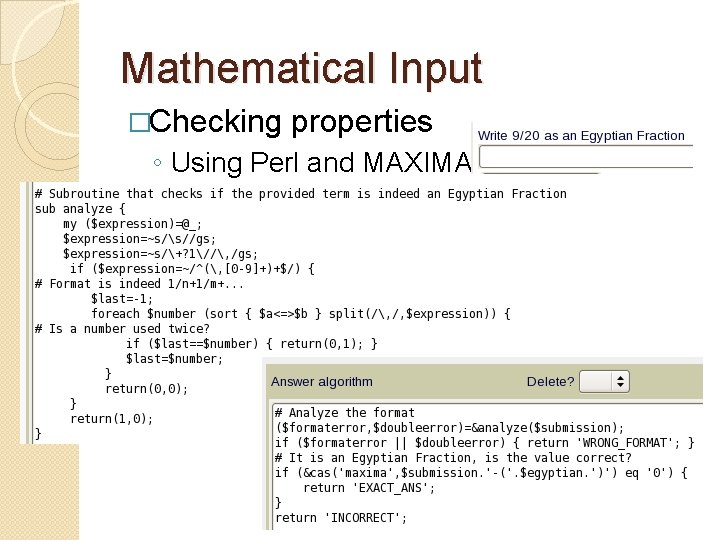Mathematical Input �Checking properties ◦ Using Perl and MAXIMA:Mathematical Input Math editor for studentsMathematical Input �Graphical input �using Geogebra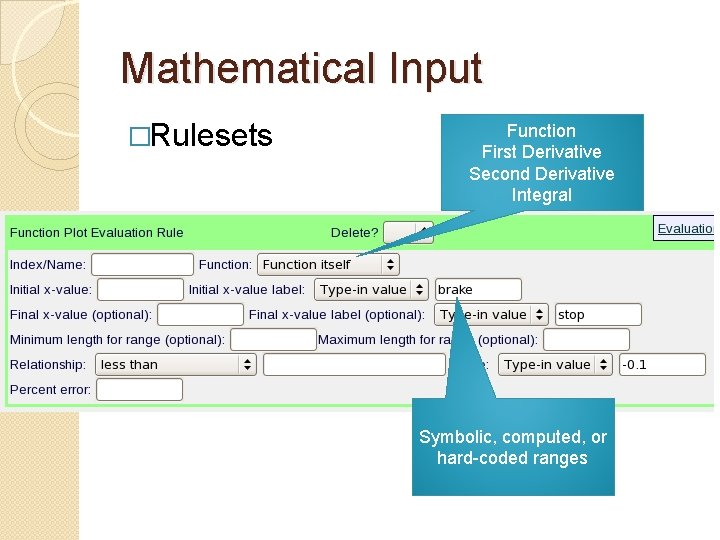Mathematical Input �Rulesets Function First Derivative Second Derivative Integral Symbolic, computed, or hard-coded ranges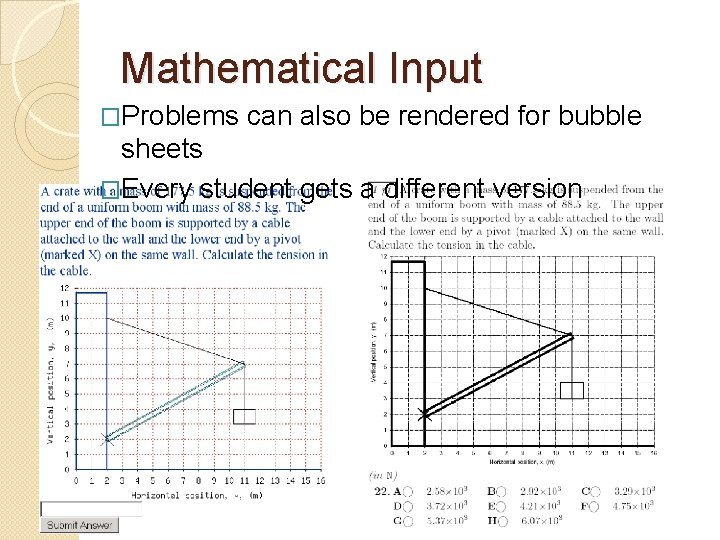Mathematical Input �Problems can also be rendered for bubble sheets �Every student gets a different versionMathematical Input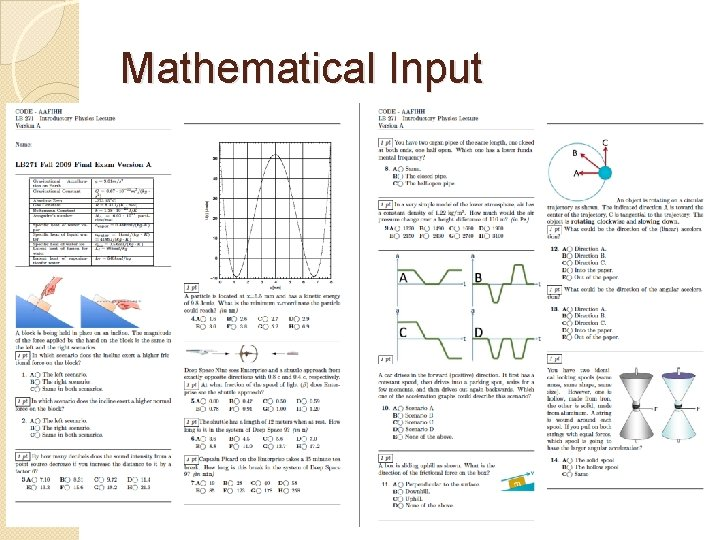Mathematical Input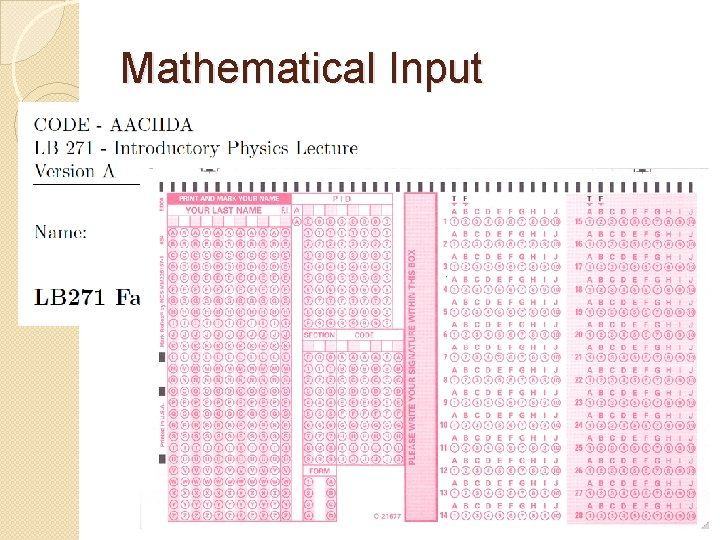Mathematical InputMathematical Input Numerical Clicker in LectureMathematical Input LON-CAPA can evaluate clicker data after lecture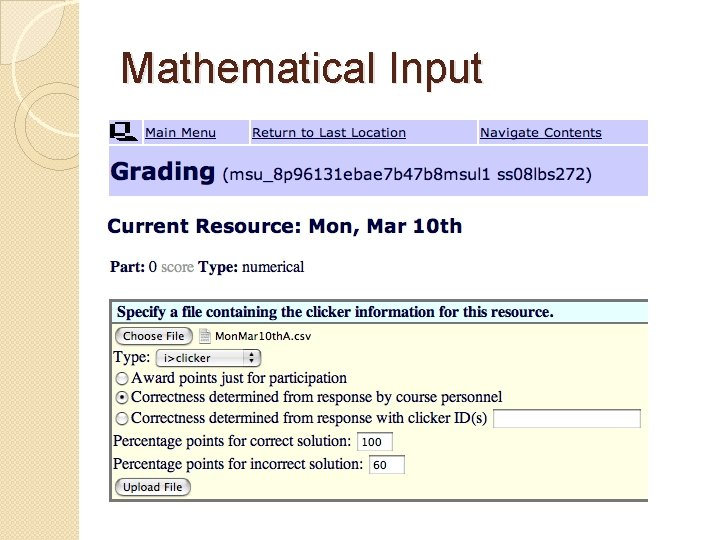Mathematical InputMathematical Input �i>clicker 2 integrate d in LONCAPAInterested? �Faculty Seminars May 10 -11, 2011 �Can give departmental colloquia �Work one-on-one �LON-CAPA Conference Virginia Commonwealth University May 19 -21, 2011 �LON-CAPA Workshop MSU, late June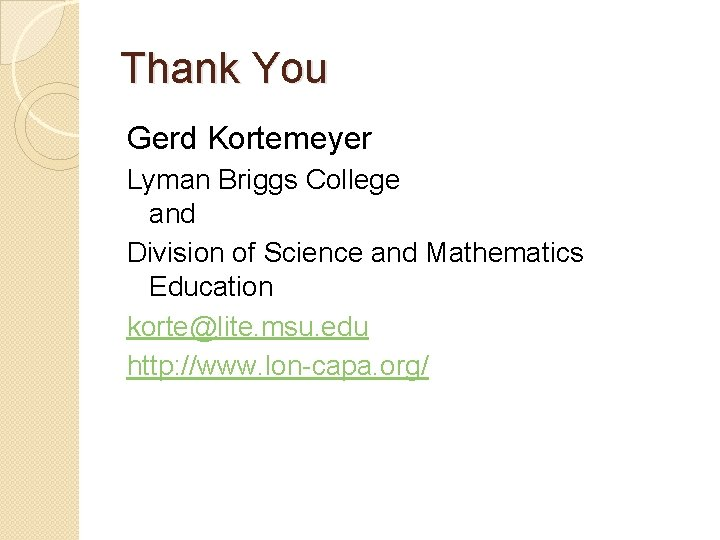Thank You Gerd Kortemeyer Lyman Briggs College and Division of Science and Mathematics Education [email protected] msu. edu http: //www. lon-capa. org/